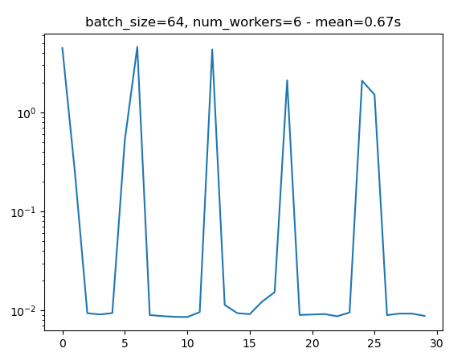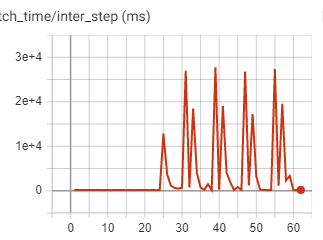# NumpyDataset - Performance Analysis

Hi everyone,

I have a directory with numpy files, each containing a data instance (for now, this image is generated randomly, without post processing - in my real scenario, it will be a numpy structured array, with some preprocessing).

I have implemented a map-style pytorch dataset, which loads the right numpy file every time getitem is called.
To avoid having to use shuffle=True (which shuffles the iterator), I have permuted the indices and saved them as an attribute. This had no effect on the performances.

I have created different dataloaders with various arguments passed (num_workers, batch_size, and etc.), and I have measured the performance across 30 batches, trying to see how the number of iterations/second changes with different parameters.

The two weird phenomena I see are:

1. Inconsistency: Sometimes, the exact code runs significantly (x17) faster on the 2nd run than on the 1st run. This is terribly weird, and I have no idea what causes this.
2. Periodical slowing: I noticed that when using num_workers=x, every xth iteration is about 10 times as slow as previous ones.

I would love it if someone could tell me why I see this behaviour and what I can do to overcome it.

Attached is a minimal reproducible example and 2 figures that show the weird behaviours (notice that these two runs have periodical nature, and both are run with the same parameters).

``````
import time
import matplotlib.pyplot as plt
import numpy as np
import torch
from pathlib import Path
from tqdm import tqdm

root = Path('/home/yonatan/Desktop/NumpyDataset/data')
num_files = 6400
def generate_dataset(root, num_files):
for i in tqdm(range(N)):
mat = np.random.randint(low=0, high=255, size=(30, 300, 300))
mat = mat.astype('int8')
file_path = root / f'file_{i}.npy'
np.save(file_path, mat)

class NumpyDataset(Dataset):
def __init__(self, root, indxs):
self.root = root
self.indices = list(indxs)
np.random.seed(0)
self.permuted_indices = np.random.permutation(self.indices)

def __len__(self):
return len(self.indices)

def __getitem__(self, idx):
permuted_index = self.permuted_indices[idx]

def measure_timing(N, batchsize, num_workers):
numpy_dataset = NumpyDataset(root=root, indxs=range(num_files))
batch_size=batchsize,
shuffle=False,
num_workers=num_workers,
pin_memory=False)
timings = [time.time()]
pbar = tqdm(total=N)
for i in range(N):
batch = next(numpy_iter)
timings.append(time.time())
pbar.update(1)
return np.diff(timings)

def measure_timing_and_plot_results(N, batch_size, num_workers):
timings = measure_timing(N=N, batchsize=batch_size, num_workers=num_workers)
total_mean = np.mean(timings)
plt.title(f'batch_size={batch_size}, num_workers={num_workers} - mean='
f'{total_mean:.2f}s')
plt.plot(timings)
plt.yscale('log')
plt.show()

if __name__ == '__main__':
# generate_dataset(root=root)
measure_timing_and_plot_results(N=30, batch_size=128, num_workers=6)
``````The periodic slowdown is most likely caused, if your data loading pipeline is too slow for the training loop.
In fact it doesn’t seem as if you are profiling the `DataLoader` in a real use case (with model training), but as a standalone application.

If you are using multiple workers, each process will load a batch and add it to the queue.
Once a batch is ready to be consumed, the `DataLoader` loop moves forward and the model training can be performed. In the background all workers will load the next batch (the number of prefetched batches is defined by the `prefetch_factor` and is set to `2*num_workers` by default).
If all workers are able to load a batch in approx. the same amount of time, the `DataLoader` loop would have `num_workers` batches to consume.
Since you don’t have any workload in this loop (again, no model training), the script just executes the loop and has to wait until the next batch is ready, i.e. until one of the workers has loaded a complete batch, and the slowdown is visible.

1 Like

Thanks for the reply, @ptrblck , I’ve learned a lot from reading it.
However, after profiling my code (with model training), I’ve still witnessed the same thing.
I used tensorboard together with pytorch lightning’s profiler to measure the inter step time (the time between one step’s end, and another’s start).
The weird behavior persists. It starts a bit after step 20, and then you see periodical slows in the fetching of data, with num_workers=8 being the period time.My Full dataset code is:

``````
class NumpyDataset(torch.utils.data.Dataset):
"""A pytorch dataset based on a folder of npy files"""
def __init__(self, root, families, chroms, indices,
transform=None):
self.root = Path(root)

self.npy_files_args = list(itertools.product(families, chroms, indices))
self.num_files = len(self.npy_files_args)
# we define the transform attribute
self.transform = transform

# # we set a pseudo-random permutation
np.random.seed(0)
self.random_permutation = np.random.permutation(self.num_files)

def get_file_path(self, index):
fam, chrom, idx = self.npy_files_args[index]
return self.root / fam / chrom / f'{fam}_{chrom}_{idx:05d}.npy'

def __getitem__(self, index):
# we use the given index to extract the sample at the permuted index
permuted_idx = self.random_permutation[index]

# except:
#     print(f'Failed to load file {self.get_file_path(permuted_idx)}')
#     return None

# if transform is given, we apply it
if self.transform:
sample = self.transform(sample)

return sample

def __len__(self):
return self.num_files
``````

If anyone knows why this behavior persists, I would love to know.
P.S The data consists of a 3d tensor + 2d tensor + little numpy arrays with some metadata information. Might the transformations on this data be heavy for the dataloaders?

You would always see the periodic peak in the data loading, if the time of the training loop is less than the data loading time for the next batch.

Yes, the transformations would cost some time, but they are not necessarily the bottleneck of the data loading pipeline and you would have to profile it.
E.g. loading the data could be slow in case you are using an HDD instead of an SSD etc.
This post explains potential bottlenecks and some workarounds.